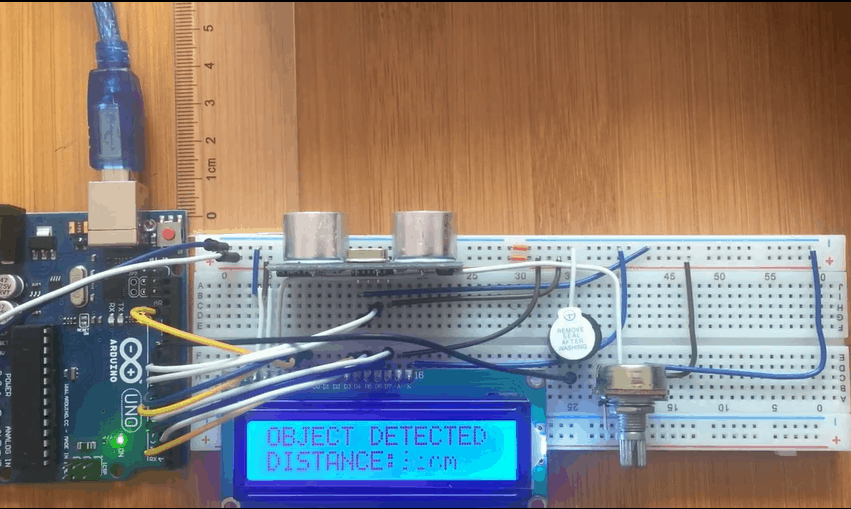# How to measure distance using Ultrasonic Sensor HC-SR04 and ArduinoThe HC-SR04 Ultrasonic sensor  can  be used to detect objects in a range of up to 13 feet away. In this tutorial this sensor is connected to Arduino to achieve this function. Ultrasound is high-pitched sound waves with frequencies higher than the audible limit of human hearing.

Human ears can hear sound waves that vibrate in the range from about 20Hz to about 20,000Hz times a second. However, ultrasound has a frequency of over 40,000 Hz and is therefore inaudible to humans.

## How the HC-sr04 ultrasonic sensor works

The HC-SR04 Ultrasonic distance sensor consists of two ultrasonic transducers. The one acts as a transmitter which converts electrical signal into 40 KHz ultrasonic sound pulses.

The receiver listens for the transmitted pulses. If it receives them it produces an output pulse whose width can be used to determine the distance the pulse travelled.

The sensor is small, easy to use in any robotics project and offers excellent non-contact range detection between 2 cm to 400 cm with an accuracy of 3mm. Since it operates on 5 volts, it can be hooked directly to an Arduino or any other 5V logic microcontrollers.

The HC-SR04 Ultrasonic Module has 4 pins, Ground, VCC, Trig and Echo. The Ground and the VCC pins of the module needs to be connected to the Ground and the 5 volts pins on the Arduino Board respectively and the trig and echo pins to any Digital I/O pin on the Arduino Board.

In this project the ultrasonic sensor is connected to an LCD screen where the distance of the object can be displayed. An buzzer is also included to sound an alarm when the object goes beyond the required distance from the reference point.

## Schematic for measuring distance with HC-SR04 Ultrasonic sensor and Arduino.

### Code for measuring distance using HC-SR04 Ultrasonic sensor and Arduino.

The code below involves triggering the ultrasonic sensor and measuring the received signal pulse width manually,

``````#include<LiquidCrystal.h>
#define trigPin 9
#define echoPin 8
#define buzzer 10
int sound=500; // variable for buzzer sound
LiquidCrystal lcd(6,7,2,3,4,5);
void setup()
{
Serial.begin (9600);
pinMode(trigPin, OUTPUT);
pinMode(echoPin, INPUT);
pinMode(buzzer, OUTPUT);
lcd.begin(16,2);
}
void loop()
{
// controlling ultrasonic sensor
long duration, distance;
digitalWrite(trigPin, LOW);
delayMicroseconds(2);
digitalWrite(trigPin, HIGH);
delayMicroseconds(10);
digitalWrite(trigPin, LOW);
duration = pulseIn(echoPin, HIGH);
distance = (duration/2) / 29.1;
//controlling buzzer
if (distance > 5)
{ noTone(buzzer); // buzzer off
}
else
{
tone(buzzer,sound); // buzzer on
}
// controlling lcd display
if (distance >= 20)
{
lcd.setCursor(0,0); //set cursor at column 0 row 1
lcd.print("NOTHING IN RANGE");
lcd.setCursor(0,2); //set cursor at column 0 row 2
lcd.print("                ");
}
else if(distance <20 || distance>5)
{
lcd.setCursor(0,0);
lcd.print("OBJECT DETECTED ");
lcd.setCursor(0,2);
lcd.print("DISTANCE:");
lcd.print(distance);
lcd.print( "cm");
}
if(distance<=5)
{
lcd.setCursor(0,0);
lcd.print("OBJECT TOO CLOSE");
lcd.setCursor(0,2);
lcd.print("DISTANCE:");
lcd.print(distance);
lcd.print( "cm");
}
delay(1000);
}
``````

The above program can be made more accurate using the NewPing library. This library contains commands that enable us to run up to 15 ultrasonic sensors at once and it can directly output in centimetres, inches or time duration.

The code below gives an example of the ultrasonic sensor controlled using the NewPing library. Here we are using the Serial Monitor to display Range Finder distance readings.

``````// Include NewPing Library
#include "NewPing.h"
// Hook up HC-SR04 with Trig to Arduino Pin 9, Echo to Arduino pin 10
#define TRIGGER_PIN 9
#define ECHO_PIN 10
// Maximum distance we want to ping for (in centimeters).
#define MAX_DISTANCE 400
// NewPing setup of pins and maximum distance.
NewPing sonar(TRIGGER_PIN, ECHO_PIN, MAX_DISTANCE);
float duration, distance;
void setup()
{
Serial.begin(9600);
}
void loop()
{
// Send ping, get distance in cm
distance = sonar.ping_cm();
// Send results to Serial Monitor
Serial.print("Distance = ");
if (distance >= 400 || distance <= 2)
{
Serial.println("Out of range");
}
else
{
Serial.print(distance);
Serial.println(" cm");
}
delay(500);
}
``````

## Displaying Distance from HC-SR04 Ultrasonic sensor on LCD as a Bar Graph.

We can now be able to display distance on LCD using a bar graph as shown in the picture below. In this case am going to use an I2C LCD.

The setup will be as shown below Trig and Echo pins of the Ultrasonic sensor are connected to Arduino digital pins 9 and 10 respectively. The SCL and SDA pins of the I2C display are connected to Arduino analog pins A5 and A4 respectively.

The major challenge with this setup is that we are using an I2C display but most Arduino LCD Bar Graph libraries do not support this type of display. In this case you need to create your own library that supports this display.

In the code below I have used a custom library called LcdBarGraph.h. You can watch the video tutorial below to learn how you can create your own library.

``````#include<Wire.h>
#include<LiquidCrystal_I2C.h>
#include<LcdBarGraphI2C.h>
LiquidCrystal_I2C lcd(0x27, 2, 1, 0, 4, 5, 6, 7, 3, POSITIVE);
LcdBarGraphI2C lbg(&lcd, 16, 0, 1); // Creates an LCD Bargraph object.
const int trigPin = 9;
const int echoPin = 10;
long duration;
int distance;
#define max_distance 30  // Maximum distance we want to ping for (in centimeters).
void setup()
{
lcd.begin(16,2); // Initializes the interface to the LCD screen
lcd.backlight();
pinMode(trigPin, OUTPUT);
pinMode(echoPin, INPUT);
}
void loop()
{
// Write a pulse to the HC-SR04 Trigger Pin
digitalWrite(trigPin, LOW);
delayMicroseconds(2);
digitalWrite(trigPin, HIGH);
delayMicroseconds(10);
digitalWrite(trigPin, LOW);
// Measure the response from the HC-SR04 Echo Pin
duration = pulseIn(echoPin, HIGH);
// Determine distance from duration
// Use 343 metres per second as speed of sound
distance= duration*0.034/2;
// Prints "Distance: " on the first line of the LCD
lcd.setCursor(0,0);
lcd.print("Distance: ");
lcd.print(distance);
lcd.print(" cm");
// Draws bargraph on the second line of the LCD
lcd.setCursor(0,1);
lbg.drawValue(distance, max_distance);
delay(500);
}
``````